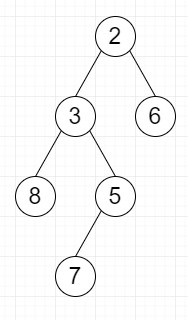# Find if given vertical level of binary tree is sorted or not in Python

Suppose we have binary tree; we have to check whether the given vertical level of the binary tree is sorted or not. When two nodes are overlapping, check they are in sorted sequence in the level they belong.

So, if the input is like l = -1then the output will be True, as elements in level -1 are 3,7 and they are sorted.

To solve this, we will follow these steps −

• if root is null, then

• return True

• previous_value := -inf

• current_level := 0

• current_node := a new tree node with value 0

• Define one dequeue named q

• insert (root, 0) at the end of q

• while q is not empty, do

• current_node := q[0, 0]

• current_level := q[0, 1]

• delete element from left of q

• if current_level is same as level, then

• if previous_value <= current_node.val, then

• previous_value := current_node.val

• otherwise,

• return False

• if current_node.left is not-null, then

• insert (current_node.left, current_level - 1) at the end of q

• if current_node.right is non-null, then

• insert (current_node.right, current_level + 1) at the end of q

• return True

## Example

Let us see the following implementation to get better understanding −

Live Demo

from collections import deque
from sys import maxsize
INT_MIN = -maxsize
class TreeNode:
def __init__(self, key):
self.val = key
self.left = None
self.right = None
def are_elements_sorted(root, level):
if root is None:
return True
previous_value = INT_MIN
current_level = 0
current_node = TreeNode(0)
q = deque()
q.append((root, 0))
while q:
current_node = q
current_level = q
q.popleft()
if current_level == level:
if previous_value <= current_node.val:
previous_value = current_node.val
else:
return False
if current_node.left:
q.append((current_node.left, current_level - 1))
if current_node.right:
q.append((current_node.right, current_level + 1))
return True
root = TreeNode(2)
root.left = TreeNode(3)
root.right = TreeNode(6)
root.left.left = TreeNode(8)
root.left.right = TreeNode(5)
root.left.right.left = TreeNode(7)
level = -1
print(are_elements_sorted(root, level))

## Input

root = TreeNode(2) root.left = TreeNode(3) root.right = TreeNode(6)
root.left.left = TreeNode(8) root.left.right = TreeNode(5)
root.left.right.left = TreeNode(7)

## Output

True